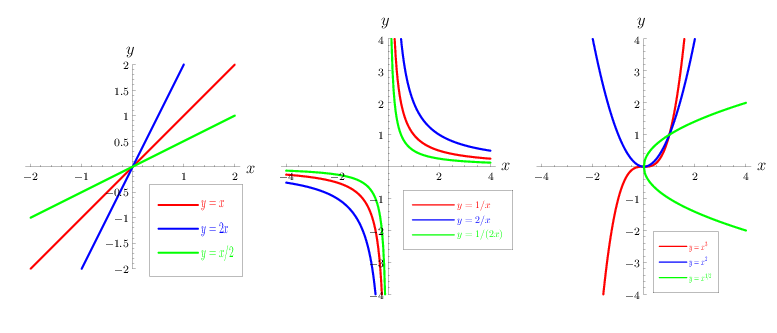# Power function

A function $f : x \mapsto y$ with

$$y = x ^ {a} ,$$

where $a$ is a constant number. If $a$ is an integer, the power function is a particular case of a rational function. When $x$ and $a$ have complex values, the power function is not single valued if $a$ is not an integer.

For fixed real $x$ and $a$, the number $x ^ {a}$ is a power, and the properties of $y = x ^ {a}$ therefore follow from the properties of the power.

When $x > 0$, the power function $x ^ {a}$ is defined and positive for any real $a$. When $x \leq 0$, the power function $x ^ {a}$ is defined in the following cases.

a) When $x= 0$, the power function $x ^ {a}$ is defined to equal 0 if $a > 0$, and is not defined if $a < 0$. The power function $x ^ {0}$ is defined to equal 1 for all $x$; in particular, $0 ^ {0} = 1$.

b) If $n$ is a natural number, then the power function $x ^ {n}$ is defined for all $x$, and the power function $1/x ^ {n} = x ^ {-} n$ is defined for all $x \neq 0$. Here $x ^ {1} = x$ and $x ^ {n} = x \cdot x ^ {n-} 1$ if $n> 1$.

c) The power function $x ^ {1/n}$, where $n$ is an odd natural number, is defined for all real $x$, and is negative when $x < 0$. However, it is sometimes convenient to restrict in this case the power function $x ^ {1/n}$ to $x \geq 0$. The same statements apply for the power function $x ^ {m/n}$, when $m/n$ is an irreducible fraction. Here $( x ^ {1/n} ) ^ {n} = x$ and $x ^ {m/n} = ( x ^ {1/n} ) ^ {m}$.The properties of $x ^ {a}$ are usually considered when $x > 0$ and $a$ is real, although many of them also hold when $x \leq 0$ and, for example, $a$ is a natural number.

Functions of the form $y = cx ^ {a}$, where $c$ is a constant coefficient and $a= 1$, express a direct proportionality (their graphs are straight lines passing through the origin of the coordinates (Fig.a)), while when $a=- 1$, they express an inverse proportionality (their graphs are equilateral hyperbolas with their centre at the origin of the coordinates and having the coordinate axes as their asymptotes (Fig.b)). Many laws of physics can be mathematically expressed by using functions of the form $y = cx ^ {a}$( Fig.c).

When $x > 0$, the power function $x ^ {a}$ is continuous, monotone (increasing when $a > 0$, decreasing when $a < 0$), infinitely differentiable, and, in a neighbourhood of every positive $x _ {0}$, can be expanded into a Taylor series. Moreover,

$$( x ^ {a} ) ^ \prime = ax ^ {a-} 1 ,$$

$$\int\limits x ^ {a} dx = \frac{x ^ {a+} 1 }{a+} 1 + C \ \textrm{ when } a \neq - 1,$$

$$\int\limits \frac{dx}{x} = \mathop{\rm ln} | x | + C,$$

$$x ^ {a} = \sum _ { n= } 0 ^ \infty \left ( \begin{array}{c} a \\ n \end{array} \right ) x _ {0} ^ {a-} n ( x- x _ {0} ) ^ {n} ,$$

when $| x- x _ {0} | < | x _ {0} |$, where $( {} _ {n} ^ {a} )$ are the binomial coefficients.

In the complex domain, the power function $z ^ {a}$ is defined for all $z \neq 0$ by the formula

$$\tag{* } z ^ {a} = e ^ {a \mathop{\rm Ln} z } = \ e ^ {a( \mathop{\rm ln} | z | + i \mathop{\rm arg} z+ 2k \pi i) } ,$$

where $k = 0, \pm 1 ,\dots$. If $a$ is an integer, then $z ^ {a}$ is single valued:

$$z ^ {a} = | z | ^ {a} e ^ {ia \mathop{\rm arg} z } .$$

If $a$ is rational ( $a = p/q$, where $p$ and $q$ are relatively prime), then the power function $z ^ {a}$ takes $q$ different values:

$$( z ^ {a} ) _ {k} = | z | ^ {a} e ^ {ia \mathop{\rm arg} z } \epsilon _ {k} ,$$

where $\epsilon _ {k} = e ^ {2k \pi i/q }$ are the $q$- th roots of unity: $\epsilon _ {k} ^ {q} = 1$ and $k = 0 \dots q- 1$. If $a$ is irrational, then $z ^ {a}$ has an infinite number of values: the factor $e ^ {a2k \pi }$ takes different values for different $k$. For non-real complex values of $a$, the power function $z ^ {a}$ is defined by the same formula (*).

Also regarding formula (*), the symbol $e ^ {w}$ is an abbreviation for the value $\mathop{\rm exp} ( w)$ of the exponential function exp at the complex number $w$. This function is defined by the series

$$\mathop{\rm exp} ( w ) = \sum _ { n= } 0 ^ \infty \frac{w ^ {n} }{n!} ,$$

which converges (absolutely) at each complex $w$. Note that $n! = w ^ {n} = 1$ if $w = n = 0$.

Taking $- \pi < \mathop{\rm arg} z \leq \pi$ and $k= 0$ in (*) one obtains the principal value. An interesting example is obtained if $z = a= i$:

$$i ^ {i} = e ^ {i \mathop{\rm Ln} i } = \ e ^ {i \cdot i \mathop{\rm arg} i } = e ^ {- \pi /2 } .$$

How to Cite This Entry:
Power function. Encyclopedia of Mathematics. URL: http://encyclopediaofmath.org/index.php?title=Power_function&oldid=48271
This article was adapted from an original article by V.I. Bityutskov (originator), which appeared in Encyclopedia of Mathematics - ISBN 1402006098. See original article